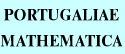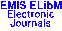PORTUGALIAEMATHEMATICA Vol. 58, No. 4, pp. 449-460 (2001)

Previous Article

Next Article

Contents of this Issue

Other Issues

ELibM Journals

ELibM Home

EMIS Home

## Nonexistence of Global Solutions of Nonlinear Wave Equations

### R. Eloulaimi and M. Guedda

Faculté des Sciences, Université Abdelmalek Essaadi,
B.P. 2121 Tétouan -- MAROC
Lamfa, CNRS UPRES--A 6119,
Université de Picardie Jules Verne, Faculté de Mathématiques et d'Informatique,
33, rue Saint-Leu 80039, Amiens -- FRANCE

Abstract: In this paper the nonexistence of global solutions to wave equations of the type $u_{tt}-\Delta u\pm u_t=\lambda\,u + \vert u\vert^{1+q}$ is considered. We derive, for an averaging of solutions, a nonlinear second differential inequality of the type $w^{\prime\prime} \pm w^\prime \geq b\,w + \vert w\vert^{1+q}$, and we prove a blowing up phenomenon under some restriction on $u(x,0)$ and $u_t(x,0)$. Similar results are given for other equations.

Full text of the article: